• 意思就是取k的二进制的最末位，11的二进制数为1011，第一次循环，取的是最右边的1，以此类推。k>>=1意思就是k右移一位，删去最低位置。 接下来我来说说while循环中的原理。 以num^11为例子，k = 11 k 的二进制 ...
最近在牛客第一次碰到有关快速幂的知识，在此记录加深理解一下。大佬勿进，我怕《《 》》
快速取幂的用途
在一些竞赛中，可能会遇到指数型的数据取模问题，这个时候直接用int或者long long 储存，就非常有可能超出计算机整数的存取范围(哈哈，一般都会超，毕竟是竞赛！！！)，从而数据出错，所以我们就引出今天的主角色 快速幂取模。这种方法在时间和空间都做了尽可能的优化，非常好用哦！
快速幂取模的思路分析
基本理论是离散数学的知识*(数学很重要!）* 有一个引理我们需要清楚的：积的取余等于取余的积的取余。 公式：(ab)%c = (a%c)(b%c)%c 核心思想是将大数幂运算拆解成相应的乘法运算，利用上述式子，始终将我们的运算的数据量控制在c的范围下。
以求a的b次方来介绍 把b转换成二进制数。 例如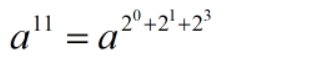11的二进制是1011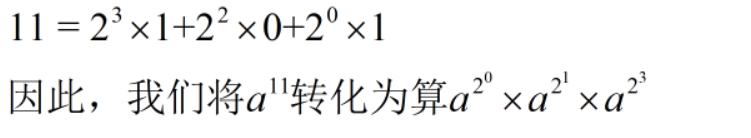对二进制的位运算，在此就不赘述，有问题的同学请自行百度，这里我们需要用到“&”与“>>”运算符。
先来一段具体代码，由代码来讲解
ll binaryPow(ll num, ll k)
{
ll ans = 1;
while(k>0)
{
if(k&1)
ans = ans*num%mod;//如果k的二进制位不是0，那么就会进行该步
num = num*num%mod;//不断加倍
k >>=1; //相当于每次除以2，用二进制看，不断的遍历k的二进制位
}
return ans;
}
在上面的代码中，k&1意思就是取k的二进制的最末位，11的二进制数为1011，第一次循环，取的是最右边的1，以此类推。k>>=1意思就是k右移一位，删去最低位置。 接下来我来说说while循环中的原理。 以num^11为例子，k = 11 k 的二进制 1011。 我们要理解num = num * num；这一步的作用。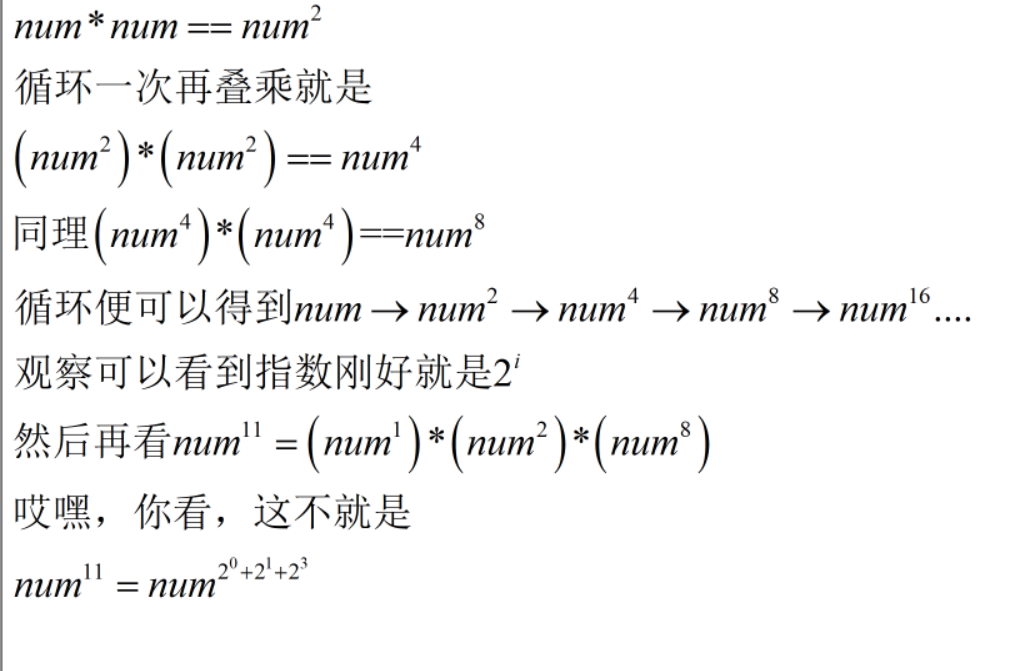哎嗨，快速幂就讲解完了。希望大家可以共同进步。另外注明一点，代码%mod也是为了防止溢出，这是从下面一道训练题想出来的。 下面贴出来一道例题。 题目链接 https://ac.nowcoder.com/acm/contest/3003/G
#include <cstdio>
#include <iostream>
#include <algorithm>
#include <cstring>
#include <cmath>
using namespace std;
typedef long long ll;
const int maxn = 200005;
const int mod = 1e9+10;
typedef long long ll;
ll binaryPow(ll num, ll k)
{
ll ans = 1;
while(k>0)
{
if(k&1)
ans = ans*num%mod;
num = num*num%mod;
k >>=1;
}
return ans;
}

int main()
{
ios::sync_with_stdio(false);
int t;
cin >> t;
while (t--)
{
long long a, b, c, d, e, f, g;
cin >> a >> b >> c >> d >> e >> f >> g;
int sum = 0;
int m = 1e9 + 7;
if (binaryPow(a,d)+binaryPow(b,e)+binaryPow(c,f) == g)
{
cout << "Yes" << endl;
}
else
{
cout << "No" << endl;
}
}
return 0;
}
就这样吧，溜走。
展开全文• 题目：来源于http://poj.org/problem?id=1060 描述：  定义二进制多项式加法和减法 ：   (x^6 + x^4 + x^2 + x + 1) + (x^7 + x + 1) = x^7 + x^6 + x^4 + x^2  (x^6
题目：来源于http://poj.org/problem?id=1060
描述：
定义二进制多项式加法和减法 ：
(x^6 + x^4 + x^2 + x + 1) + (x^7 + x + 1) = x^7 + x^6 + x^4 + x^2
(x^6 + x^4 + x^2 + x + 1) - (x^7 + x + 1) = x^7 + x^6 + x^4 + x^2
定义二进制多项式乘法：
(x^6 + x^4 + x^2 + x + 1) (x^7 + x + 1) = x^13 + x^11 + x^9 + x^8 + x^6 + x^5 + x^4 + x^3 + 1
定义二进制多项式求余数;
(x^6 + x^4 + x^2 + x + 1) (x^7 + x + 1) modulo (x^8 + x^4 + x^3 + x + 1) = x^7 + x^6 + 1  要求：输入g（x）、f（x）和h（x） 求出（g（x）*f（x））mod h（x）
具体输入要求如下：
2    （2个测试样例）
7 1 0 1 0 1 1 1   （g（x）     7表示多项式最大为x^6依次往下推）
8 1 0 0 0 0 0 1 1 （f（x） 8表示多项式最大为x^7依次往下推）
9 1 0 0 0 1 1 0 1 1 （h（x） 9表示多项式最大为x^8依次往下推）  这是第一测试样例，即每个测试样例占三行
10 1 1 0 1 0 0 1 0 0 1
12 1 1 0 1 0 0 1 1 0 0 1 0
15 1 0 1 0 1 1 0 1 1 1 1 1 0 0 1

输出结果：

8 1 1 0 0 0 0 0 1
14 1 1 0 1 1 0 0 1 1 1 0 1 0 0

思路：
（1）  乘法：定义一个bitwap，做乘法的时候，先输入g（x），然后输入f（x）。每当f（x）第n位不等于0，就将g（x）移动n位即在bitmap上从n位开始，将g（x）加到bitmap上。
（2） 除法：每次都让h（x）与bitmap上的最高位len到len-len（h）+1与，相当于乘了x^n，将h（x）的最高位系数乘到乘积的最高位，然后求出余数比较余数的最高位与h（x）的最高位谁大，如果余数的最高位小于h（x）的最高位，则结束。

代码:
#include <iostream>
#include<cstdlib>

using namespace std;

#define  bitmapLen 2000
bool bitmap[bitmapLen];
bool Fx;
bool Hx;

class Modular{
public:
Modular(int n){
this->n = n;
}
void solution()
{
int i,j,k;
for(i=0;i<n;i++){
for(j=0;j<bitmapLen;j++){
bitmap[j] = false;
}

int len1;
cin>>len1;
for(j=0;j<len1;j++){//输入g（x）
int bit1;cin>>bit1;
Fx[len1 - j-1] = (bool)bit1;
}

int len2;
cin>>len2;
int count = len2-1;
for(j=0;j<len2;j++){//求乘积
int bit2;cin>>bit2;//输入h（x），同时求乘积
if(bit2){
for(k=0;k<len1;k++)
bitmap[k+count] ^= Fx[k];
}
count--;
}

int len = len1+len2-2;

int len3;cin>>len3;
for(j=0;j<len3;j++){//输入h（x）
int bit3;cin>>bit3;
Hx[len3 - j-1] = (bool)bit3;
}
len3--;
while(len3<=len){
j = len3;
for(k=len;k>=len-len3;k--){//取商求余
bitmap[k] ^= Hx[j--];
}

while(bitmap[len]==0)len--;//得到余数的最高位
}
cout<<len+1<<" ";
for(k = len;k>=0;k--)cout<<bitmap[k]<<" ";
cout<<endl;

}
}
protected:

private:
int n;
};

int main()
{
int n;
cin>>n;
Modular poj1060(n);
poj1060.solution();
system("pause");
return 0;
}

后记：困，饿 
展开全文• 取模运算与取余运算的相同点 公式相同： 取模运算： A mod B = A - (A / B) * B 取余运算： A rem B = A - (A / B) * B 取模运算与取余运算的不同点 对于 A / B 的定义不同： 取模运算在计算 A / B 的值时，向...
取模运算与取余运算的相同点
公式相同： 取模运算： A mod B = A - (A / B) * B 取余运算： A rem B = A - (A / B) * B
取模运算与取余运算的不同点
对于 A / B 的定义不同： 取模运算在计算 A / B 的值时，向负无穷方向取整(floor()函数) 取余运算在计算 A / B 的值时，向0 方向取整(fix()函数)  举例说明： -3 / 2 = -1.5 取模运算时，将 -1.5 向负无穷方向取整，得到 -2 取余运算时，将 -1.5 向0方向取整，得到 -1 所以， -3 mod 2 = -3 - (-2) * 2 = 1 -3 rem 2 = -3 - (-1) * 2 = -1
总结
取余运算与取模运算在两数为同号时，结果相同；当两数异号时，结果不同。
展开全文java
• 好的标题就告诉我们该来的还是会来...二进制数据是用0和1两个数码来表示的。它的基数为2，进位规则是 “逢二进一”，借位规则是 “借一当二”。二进制数据是采用位置计数法，其位权是以2为底的幂。（所有的十进制...
好的标题就告诉我们该来的还是会来，学了十几年十进制现在告诉我要学二进制，但是这个东西很重要很重要，所以还是要重点记
part 1：什么是二进制呢
二进制，是计算技术中广泛采用的一种数制，由德国数理哲学大师莱布尼茨于1679年发明。二进制数据是用0和1两个数码来表示的数。它的基数为2，进位规则是 “逢二进一”，借位规则是 “借一当二”。二进制数据是采用位置计数法，其位权是以2为底的幂。（所有的十进制数字都可以用2的n次方加2的m次方加…来表示）
part 2：十进制与二进制的相互转化 十进制整数转二进制数：“除以2取余，逆序排列” 十进制小数转二进制数：“乘以2取整，顺序排列”（是不是有个疑问这样的话有的数就永远也乘不完了，是的就是乘不完，所以一般会保留小数点后几位什么的） eg： （整数）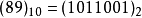89÷2 ……1 44÷2 ……0 22÷2 ……0 11÷2 ……1 5÷2 ……1 2÷2 ……0 1… … …1
二进制数转十进制数：按权展开求和 eg：这里的括号外面的数表示这是几进制的数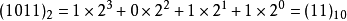（以上都是我从度娘手中得知的）
part 3：位运算 首先还是搞明白什么东西是位运算，位运算就是计算机中专门用于二进制的运算，其中包括按位与（&）、按位或（|）、按位异或（^）、按位取反（~）、按位左移（<<）、按位右移（>>）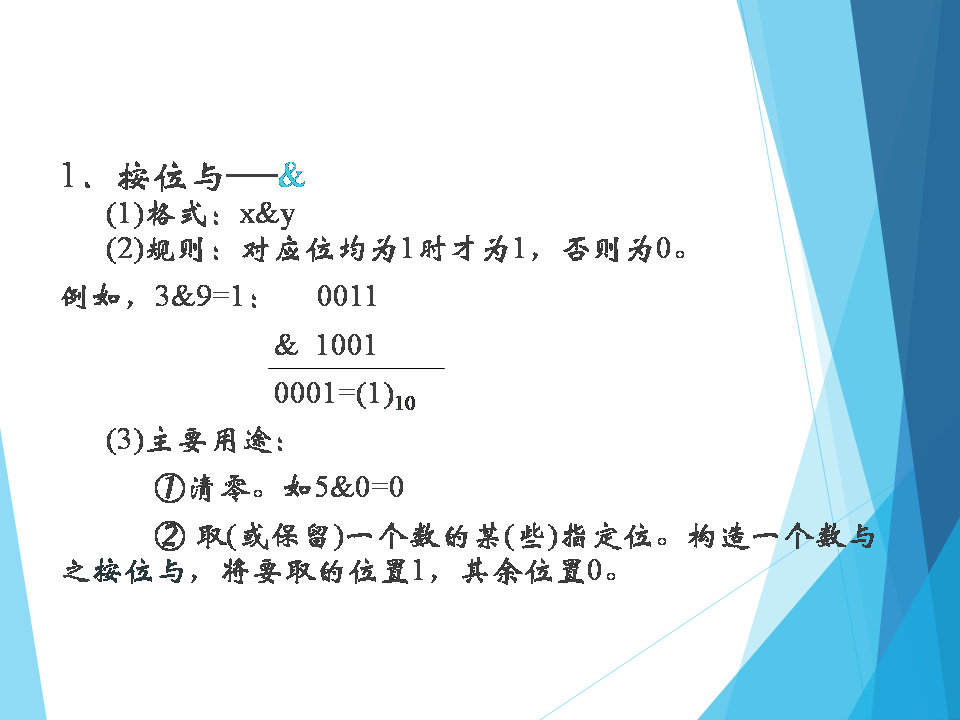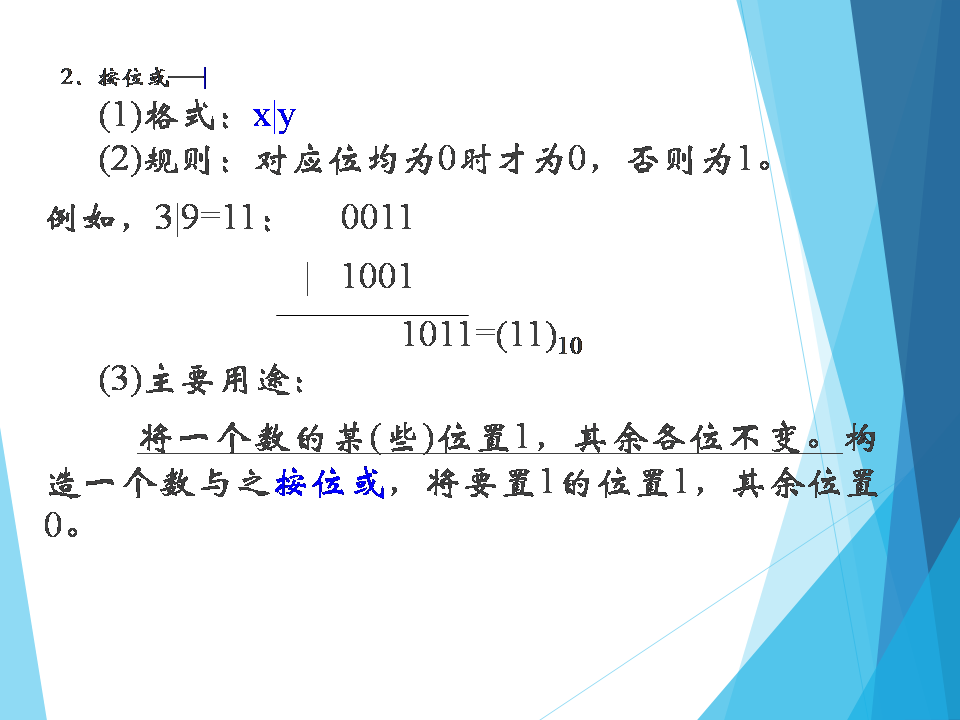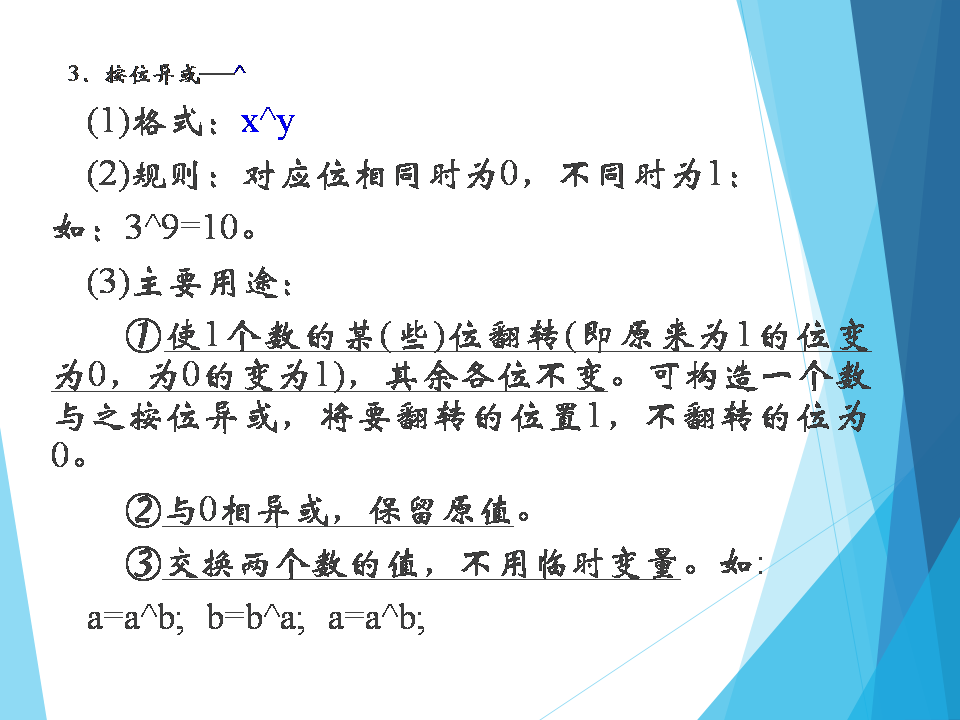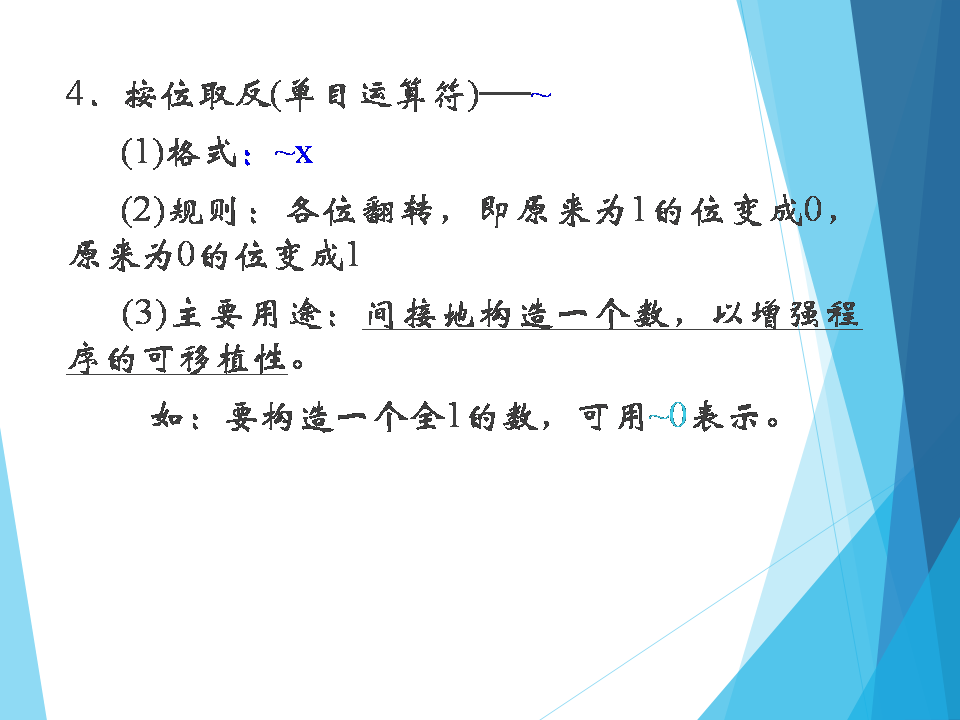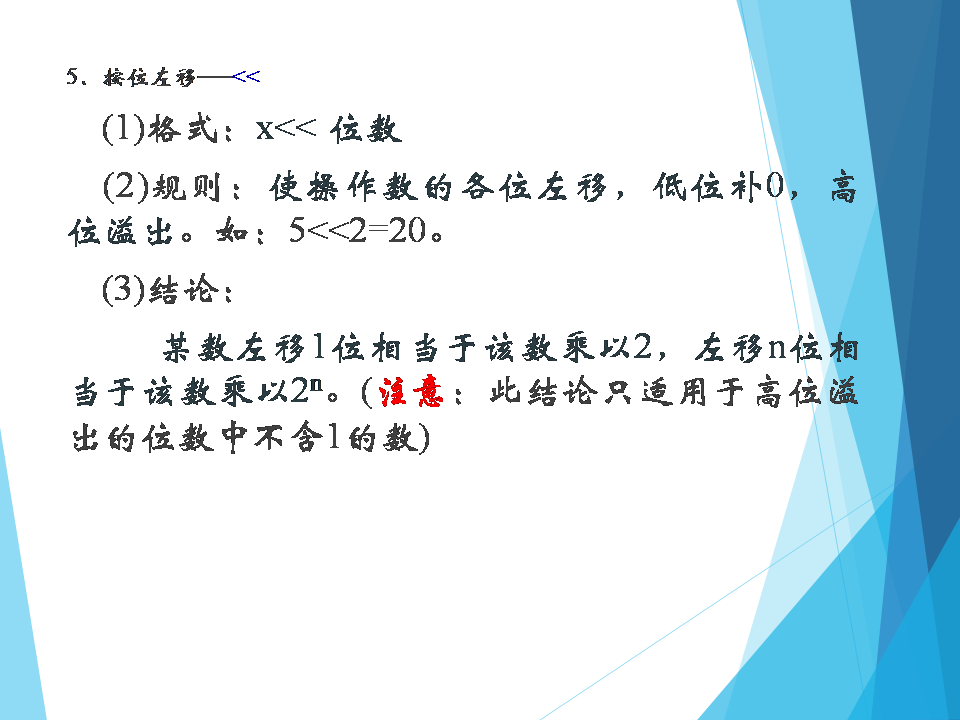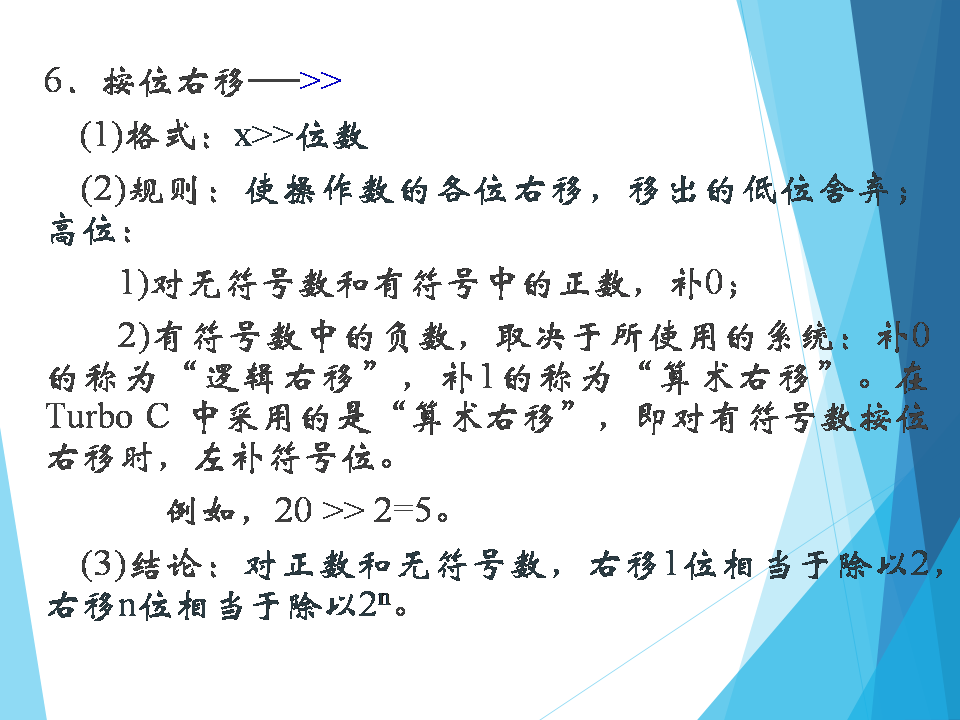以上是六个位运算符号的简单介绍（ppt是我们老师给我们上课用的咳）
位运算符的优先级顺序（由高到低）（其实可以不记，用的时候打括号就好了）part 4：快速幂 说了这么久终于到了正题，那么什么是快速幂呢，我们知道要求一个数的n次方可以用for循环不断的累积，但是这个所需要的时间是很长的（咱也没学过咱也不敢说），如果用快速幂可以节省很多时间的，所以快速幂就是一个用于很快的求一个数的n次方的东西。 快速幂有好多好多版本，以下是我们老师说的一种
int power(int a,int b)
{
int ans=1;
for(;b;b=b>>1)//这里的中间单独一个b是判断b是否不为零
{
if(b&1)ans=ans*a;
a=a*a;
}
return ans;
}

举个栗子 比如说求a的11次方，11转化成二进制是1011，那么就是a的1011次方（注意不要搞混了！是二进制中的1011）
part 1：1011&1=1；ans=a；a=a2part 2：b=101；101&1=1；ans=a 3； a=a4；part 3：b=10；10&1=0；ans=a3；a=a8；part 4：b=1；1&1=1；ans=a11；a=a22；part 5：return ans；
快速幂是个令人头秃的东西，虽然也不是很难理解，但要注意有的地方特别的bt……题目中用的时候会用^+数字来表示这个数的n次方，但是 ^这个东西也是按位异或的符号，所以要特别看清（按位异或只在二进制的计算中有） 取余运算是快速幂的一种应用（好像要用到数论的东西但是我不会所以就先不说啦），其实只要在每个算式后面加上取模就可以防止在运算过程中数字超出长整型的范围
#include<iostream>
using namespace std;
long long p,b,k,s;
long long power(long long b,long long p,long long k)
{
long long ans=1%k;
for(;p;p>>=1)
{
if(p&1)ans=ans*b%k;
b=b*b%k;
}
return ans;
}
int main()
{
cin>>b>>p>>k;
s=power(b,p,k);
cout<<b<<"^"<<p<<" mod "<<k<<"="<<s;
return 0;
}

这是一道来自洛谷的模板题 点此进入 啊大概就是这样了叭qvq
展开全文c++
• 描述：m,n,k,为整数，求 (m^n)%k=？ 正经代码： #include&lt;stdio.h&gt; using namespace std; int main(){ int m,n,k; scanf("%d%d%d",&amp;...=1,m=(long long)m...模板
• 文章目录十进制转二进制机器与真值原码、反码、补码顺便说一说BCD码数的定点表示与浮点表示IEEE 754标准定点运算加法与减法运算溢出浮点运算加法与减法运算 十进制转二进制 正整数转二进制，这个简单，除2取余，倒...
•   #include void main() ... printf(" 齿轮不加1");... printf(" 运转齿轮%d",d); }   if(c>2) {  printf("齿轮需要加1");  printf(" 运转齿轮 %d",d+1); }   }
• 进制转换：二进制、八进制、十六进制、十进制之间的转换 不同进制之间的转换在编程中经常会用到，尤其是C语言。 将二进制、八进制、十六进制转换为十进制 二进制、八进制和十六进制向十进制转换都非常容易，就是...
• 1.将二进制数转换成十进制 转换规则： 展开位权进行求和运算 100110 1x2^5+0x2^4+0x2^3+1x2^2+1x2^1+0x2^0 1x32+0x16+0x8+1x4+1x2+0x1 32+0+0+4+2+0 结果=38 2.将十进制转换为二进制 转换规则：除2取余直至运算结果...
• ## 二进制运算

千次阅读 2019-03-10 16:27:06
1.十进制转化为二进制(编译器为32进制) #include&amp;amp;lt;iostream&amp;amp;gt; using namespace std; int main() { int m,number ,s; cin&amp;amp;gt;&amp;amp;gt;number; for...
• 给你一个整数 n ，请你将 1 到 n 的二进制表示连接起来，并返回连接结果对应的十进制数字对 10 ^ 9 + 7 取余的结果。 示例 1： 输入：n = 1 输出：1 解释：二进制的 "1" 对应着十进制的 1 示例 2： .
• 1.十进制转二进制：(如果是整数)除以2取余，逆序排列，(如果是小数)乘以2取整，顺序排列 例：10(10)=1010(2) 10%2=0  5%2=1  2%2=0  1%2=1 最后表示为二进制就是1010 例： (0．625)10= (0．101)2 0....
• 在正常的运算规则下，我们熟悉的十进制会转化成二进制在计算机中表示，这时的二进制就是原码表示，在计算机中，为了简化运算单元的逻辑处理、降低硬件电路复杂度和成本，只有加法器的硬件电路，计算机的减法是通过...补码
• 二进制 　正整数的二进制表示 (假定类型是byte) 　正整数的二进制表示与此类似， 只是在十进制中，每个位置可以有10个数字，从0到9，但在二进制中，每个位置只能是0或1。 　例如： 0000 1010 ==> 10 负整数...
• 椭圆曲线加密体系中二进制域内多项式基表示的求模算法解析
• 以十进制的“19”转换为二进制数为例，用19除以模（在这里模就是2）然后取它的余数。 19除以2商9余1 9除以2商4余1 4除以2商2余0 2除以2商1余0 1除以2商0余1 当商为0时结束运算 所以19的二进制为11001
• #include <iostream> using namespace std;... // 取余 n = n >> 1; //右移一位 相当于除以2 if(0 != n) { BinaryRecursion(n); } cout<<a; } int main(int argc, char *argv[])c++
• 二进制补码到十进制补码及其内的运算——关于补码的一点学习
• 十进制转换为二进制数、八进制、十六进制的方法： 二进制数、八进制、十六进制转换为十进制的方法：按权展开求和 与十进制 （1）二进制转十进制 方法：“按权展开求和” 【例】： 规律：...
• 注：部分网上查找的资料，如有侵权，请联系我...对于十进制转二进制，首先想到的就是"除2取余倒转"这种方法。稍微要注意的就是它是从低位开始，记得要倒转。  private void decimalToBinary(int n) {  ...
• 二进制加，减法 二进制最高位为1时表示负数，为0时表示正数。 **原码：**一个正数，转换为二进制位就是这个正数的原码。负数的绝对值转换成二进制位然后在高位补1就是这个负数的原码。 举例说明： 　int类型的 3 的...
• 用-O3来编译所有的软件包将产生更大体积更耗内存的二进制文件，大大增加编译失败的机会或不可预知的程序行为（包括错误）。这样做将得不偿失，记住过犹不及。在gcc 4.x.中使用-O3是不推荐的。 -Os：这个等级用来优化...编译优化
• 参与运算的两通过“异或”原则确定商的符号，再利用其绝对值相除获取商和余数。 详细步骤：对给定两X与Y，求X/Y ①初始化时，置R=X*，Q=0； ②执行R-Y，若结果大于0，在Q最低位上商1，转④； ③在Q最低位上...
• 二进制计算 每一位上的基数的索引次幂相加之和 例如：0101=12º+12²=5 第一位1基数2的索引0次幂+第三位1*基数2的2次幂等于5 其他进制计算等同 十进制转2进制：除2求余法 除2求余倒序表示 简便算法：记住2的10次...
• 在学习框架源码底层时，有非常多的二进制运算，由于大学学习计算机基础时抓梦脚（jio），没有学习牢固，所以在看底层源码的算法逻辑时遇到二进制 运算比较吃力，遂通过一篇博文来总结下二进制运算，记录一下。 正文 ...计算机基础 源码、反码、补码
• 十进制整数转换为二进制整数采用"除2取余，逆序排列"法。具体做法是：用2去除十进制整数，可以得到一个商和余数；再用2去除商，又会得到一个商和余数，如此进行，直到商为零时为止，然后把先得到的余数作为二进制数...java
• 先给大家送个福利！ ---------------简单口算-------------------------- ...十进制转二进制规则是:除二取余倒写 10 10/2 0 5/2 1 2/2 0 1 */ ------------------------------------------干货------...
• 十进制二进制数，跟据不同的开发语言其转换方式有很多，在Java中如果相把一个十进制的整数来转换成二进制那是举手之劳，非常简单，只要用Integer.toBinaryString(int)方法就可以得到结果。但如果转换的不是一个...
• 给你一个整数 n ，请你将 1 到 n 的二进制表示连接起来，并返回连接结果对应的 十进制 数字对 109 + 7 取余的结果。 示例 1： 输入：n = 1 输出：1 解释：二进制的 “1” 对应着十进制的 1 。 示例 2： 输入：n = 3 ...
• C++学习记录：将十进制转换为二进制数（补码形式） 0、以下操作前提：不动符号位 基本运算： 1、正数的补码 等于 原码； 2、负数的补码 等于 原码取反，末位再加一； 推论： 3、补码的补码 等于 原码； 4、反码的......